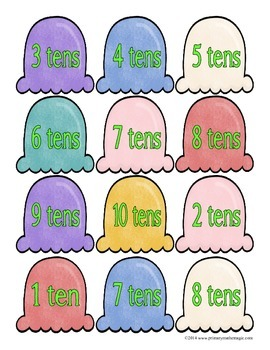# Scooping Up Tens Subtraction PackSubject
Resource Type
File Type

PDF

(4 MB|31 pages)
Standards
• Product Description
• StandardsNEW
Students practice understanding place value using tens in this 31 page pack that includes 3 different hands-on activities, reproducible sets of Base ten rods, and a label for each activity set. There are practice worksheets following each activity.

In Set 1: Scoop Up the Tens, students use ice cream scoops and cones to match different representations of multiples of tens. 1.NBT.A.1; 1.NBT.B.a; 1.NBT.B.c

In Set 2: Scoop Up Ten Less, students use ice creams scoops and cones to find 10 less than a number. 1.NBT.C.4; 1.NBT.C.5

In Set 3: Scoops of Ten, students subtract multiples of tens, matching ice cream scoops and cones. 1.NBT.C.4; 1.NBT.C.5; 1.NBT.C.6
Subtract multiples of 10 in the range 10-90 from multiples of 10 in the range 10-90 (positive or zero differences), using concrete models or drawings and strategies based on place value, properties of operations, and/or the relationship between addition and subtraction; relate the strategy to a written method and explain the reasoning used.
Given a two-digit number, mentally find 10 more or 10 less than the number, without having to count; explain the reasoning used.
Add within 100, including adding a two-digit number and a one-digit number, and adding a two-digit number and a multiple of 10, using concrete models or drawings and strategies based on place value, properties of operations, and/or the relationship between addition and subtraction; relate the strategy to a written method and explain the reasoning used. Understand that in adding two-digit numbers, one adds tens and tens, ones and ones; and sometimes it is necessary to compose a ten.
The numbers 10, 20, 30, 40, 50, 60, 70, 80, 90 refer to one, two, three, four, five, six, seven, eight, or nine tens (and 0 ones).
10 can be thought of as a bundle of ten ones - called a “ten.”
Total Pages
31 pages
N/A
Teaching Duration
N/A
Report this Resource to TpT
Reported resources will be reviewed by our team. Report this resource to let us know if this resource violates TpT’s content guidelines.